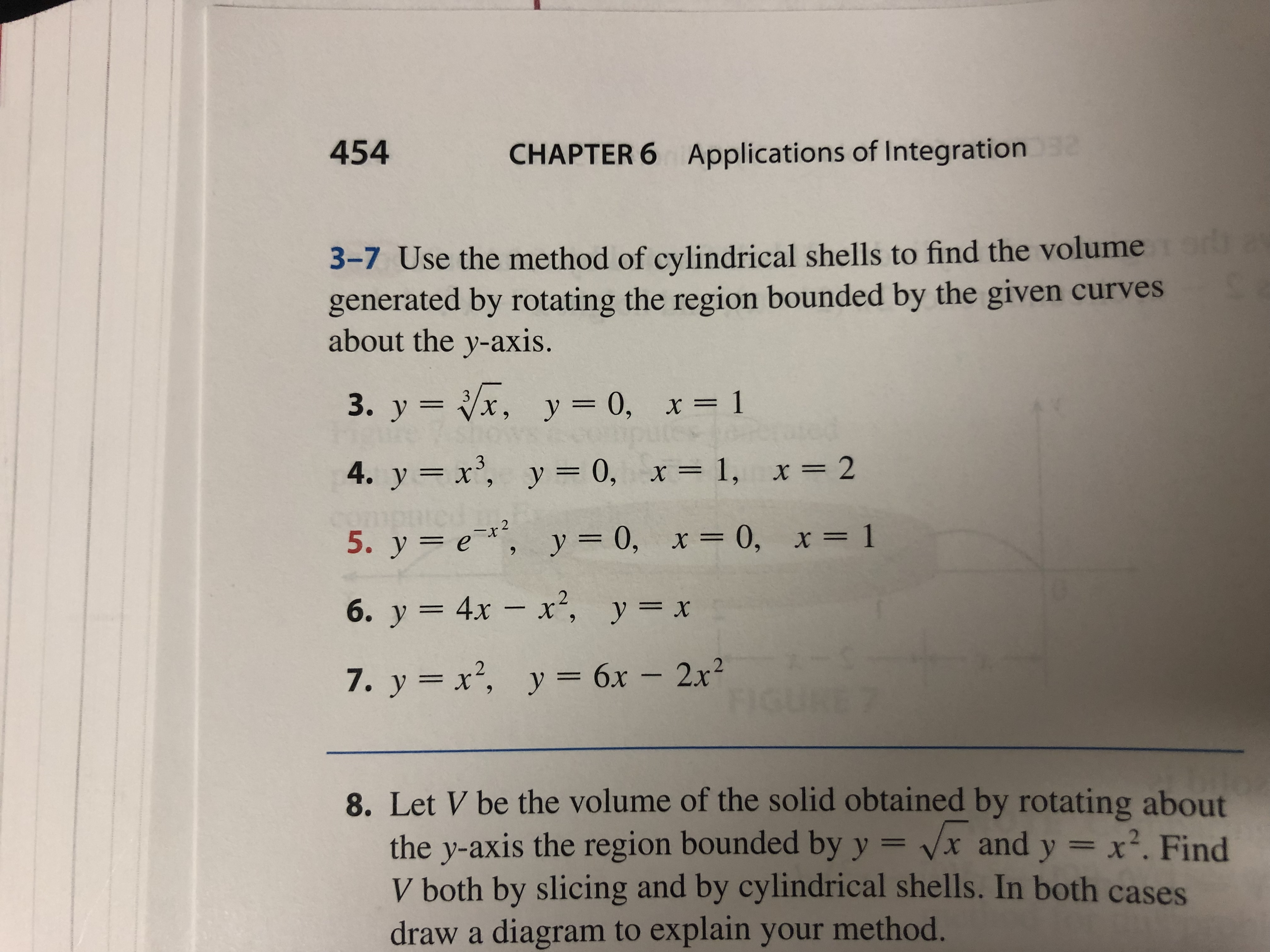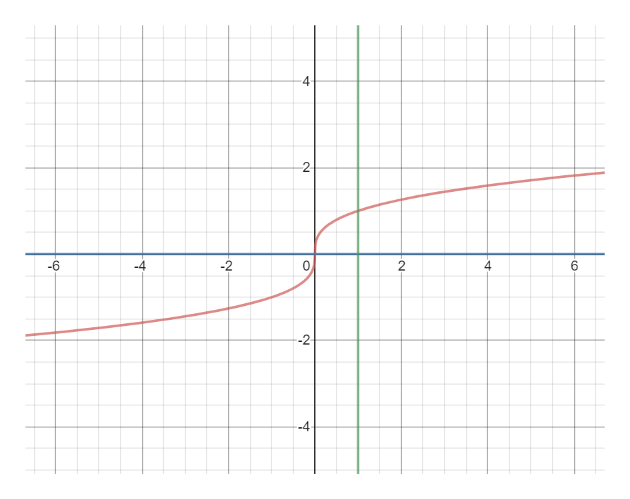454CHAPTER 6Applications of Integration23-7 Use the method of cylindrical shells to find the volumegenerated by rotating the region bounded by the given curvesabout the y-axis.3. y x, y = 0, x= 14. y x, y = 0, x= 1, x = 225. у %3D е*, у 3D0, х3D 0, х%3D 16. у 3 4х — х*, у%3D х7. y x2, y 6x - 2x28. Let V be the volume of the solid obtained by rotating aboutthe y-axis the region bounded by y = vx and y x2. FindV both by slicing and by cylindrical shells. In both casesdraw a diagram to explain your method.

Question

#3help_outlineImage Transcriptionclose454 CHAPTER 6 Applications of Integration 2 3-7 Use the method of cylindrical shells to find the volume generated by rotating the region bounded by the given curves about the y-axis. 3. y x, y = 0, x= 1 4. y x, y = 0, x= 1, x = 2 2 5. у %3D е*, у 3D0, х3D 0, х%3D 1 6. у 3 4х — х*, у%3D х 7. y x2, y 6x - 2x2 8. Let V be the volume of the solid obtained by rotating about the y-axis the region bounded by y = vx and y x2. Find V both by slicing and by cylindrical shells. In both cases draw a diagram to explain your method. fullscreen
Step 1

First graph the functions roughly...help_outlineImage Transcriptionclose-6 -4 -2 2 --2 CO 4 fullscreen

Want to see the full answer?

See Solution

Want to see this answer and more?

Our solutions are written by experts, many with advanced degrees, and available 24/7

See Solution
Tagged in

Integration